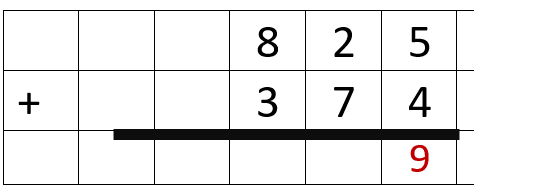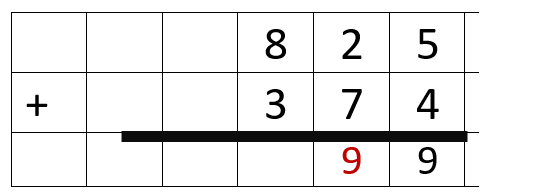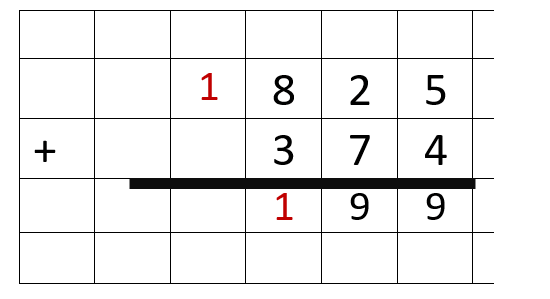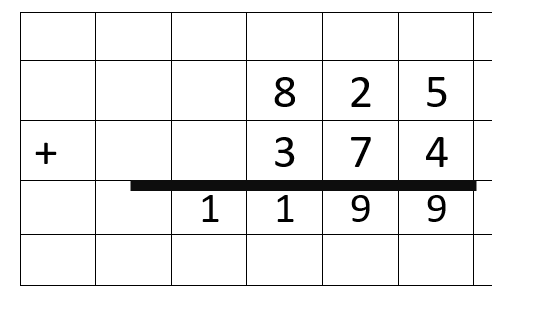#### Aligned To Common Core Standard:

When we are working on finding a sum that requires us to carry a value from one place value to another, we call this regrouping. In most cases it will be moving values of ten since it is a base ten system. You will need to become proficient with this skill as you move on to larger and even larger sums. Regrouping is used in all mathematical operations except division. This simple yet effective technique allows you to track the movement of an entire place value from one to the other. As you progress through the various type of operations you will begin to understand that values can be regrouped to the left or right. In this case, since we are focused on addition the regrouping occurs in a forward motion or right to left. This is the most basic form of regrouping which helps us prepare ourselves to perform higher levels of calculations, as we move through. In this section of our site we have fit all of the problems into a grid like matrix to allow you to easy discern the different place values.

### Printable Worksheets And Lessons

• Step-by-step Lesson- We provide with a detailed explanation about how to find the sum of 28 and 5, when it requires you to regroup in the tens column.

• Guided Lesson - This lesson is setup well because it helps students progress through single, double, and triple digit sums with this technique.

• Guided Lesson Explanation - The red text indicates the moves that you are making along the way to solve these problems. In general, they all require more than expected steps because we almost over explain what is going on.

• Practice Worksheet - All 20 problems are embedded in a graph paper, if you will, matrix which allows you to easyily align everything for yourself.

• Matching Worksheet - We really did a good job summing up all the problems into tight spaces. Which gears it more towards students that are doing well with the skill, thus far.#### Homework Sheets

We provide you with a grid style background to help you along with keeping things aligned and straight.

• Homework 1- 20 practice problems for you to get started on. They are mostly bottom heavy.
• Homework 2- These are all triple digit values with no grids to fall back on.
• Homework 3- We work on adding 3 addends here. Everything is double digits, but it is a bit complex.

#### Practice Worksheets

I enlarged the size of all of these blocks. You should be able to easily tell the place values.

• Practice 1- The bottom addend is usually greater on this sheet and that can throw students a bit.
• Practice 2- All of the answers are over a thousand.
• Practice 3- This is more of an advanced skill for students to work with.

#### Skill Quizzes

These are very helpful to see where your students are at with this skill. Each progression is tougher than the last.

• Quiz 1- We break the grid out just one last time.
• Quiz 2- This is where we work with some sizable addends and final sums.
• Quiz 3- This is, by far, where students have some trouble. Just remind them of all the great techniques that they have reviewed.

### How Do You Add and Regroup?

Let's take a look at the problem below.Step 1) Add the ones column (5 + 4 = 9).
Step 2) Write the 9 in the ones column answer.Step 3) Add the tens column (2 + 7 = 9).
Step 4) Write the 9 in the tens column answer.Step 5) Add the hundreds column (8 + 3 = 11).
Step 6) 11 = 1 thousand and 1 hundred Regroup the 1 in the thousands column. Write the 1 in the hundreds column answer.
Step 7) Write the 1 in the thousands column answer.